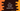# C++ program to find the square root of a number by using sqrt## How to use sqrt method to find the square root of a number in C++:

math.h header file or cmath provides a couple of useful utility mathematical functions. For finding the square root of a number, we can easily use sqrt function. This function takes one number as its parameter and returns the square root for that number. In this post, I will show you how to use sqrt method with an example.

## Definition of sqrt:

sqrt is defined as like below:

``````float sqrt(float num)
double sqrt(double num)
long double sqrt(long double num)``````

As you can see here, it takes one parameter, i.e. the number to find the square root.

To use this, we need to import cmath or math.h header file.

## Example program:

Let’s take a look at the example program:

``````#include <iostream>
#include <math.h>
using namespace std;

int main()
{
float num;

cout << "Enter a number: " << endl;
cin >> num;

cout << "Square root: " << sqrt(num) << endl;

return 0;
}``````

This program takes one number as the input from the user and finds the square root. If you run this program, it will print output as like below:

``````Enter a number:
111
Square root: 10.5357

Enter a number:
100
Square root: 10``````

## Finding square root of all numbers in a range(1 to 100):

We can also find the square roots of all numbers in a range. We need to run one loop for that. For example, the below program uses one for loop to find the square root of all numbers from 1 to 100.

``````#include <iostream>
#include <math.h>
using namespace std;

int main()
{
for (int i = 1; i <= 100; i++)
{
cout << "Square root for " << i << ": " << sqrt(i) << endl;
}
return 0;
}``````

It will find the square root of all numbers from 1 to 100 and print them.

``````Square root for 1: 1
Square root for 2: 1.41421
Square root for 3: 1.73205
Square root for 4: 2
Square root for 5: 2.23607
Square root for 6: 2.44949
Square root for 7: 2.64575
Square root for 8: 2.82843
Square root for 9: 3
Square root for 10: 3.16228
Square root for 11: 3.31662
Square root for 12: 3.4641
Square root for 13: 3.60555
Square root for 14: 3.74166
Square root for 15: 3.87298
Square root for 16: 4
Square root for 17: 4.12311
Square root for 18: 4.24264
Square root for 19: 4.3589
Square root for 20: 4.47214
Square root for 21: 4.58258
Square root for 22: 4.69042
Square root for 23: 4.79583
Square root for 24: 4.89898
Square root for 25: 5
Square root for 26: 5.09902
Square root for 27: 5.19615
Square root for 28: 5.2915
Square root for 29: 5.38516
Square root for 30: 5.47723
Square root for 31: 5.56776
Square root for 32: 5.65685
Square root for 33: 5.74456
Square root for 34: 5.83095
Square root for 35: 5.91608
Square root for 36: 6
Square root for 37: 6.08276
Square root for 38: 6.16441
Square root for 39: 6.245
Square root for 40: 6.32456
Square root for 41: 6.40312
Square root for 42: 6.48074
Square root for 43: 6.55744
Square root for 44: 6.63325
Square root for 45: 6.7082
Square root for 46: 6.78233
Square root for 47: 6.85565
Square root for 48: 6.9282
Square root for 49: 7
Square root for 50: 7.07107
Square root for 51: 7.14143
Square root for 52: 7.2111
Square root for 53: 7.28011
Square root for 54: 7.34847
Square root for 55: 7.4162
Square root for 56: 7.48331
Square root for 57: 7.54983
Square root for 58: 7.61577
Square root for 59: 7.68115
Square root for 60: 7.74597
Square root for 61: 7.81025
Square root for 62: 7.87401
Square root for 63: 7.93725
Square root for 64: 8
Square root for 65: 8.06226
Square root for 66: 8.12404
Square root for 67: 8.18535
Square root for 68: 8.24621
Square root for 69: 8.30662
Square root for 70: 8.3666
Square root for 71: 8.42615
Square root for 72: 8.48528
Square root for 73: 8.544
Square root for 74: 8.60233
Square root for 75: 8.66025
Square root for 76: 8.7178
Square root for 77: 8.77496
Square root for 78: 8.83176
Square root for 79: 8.88819
Square root for 80: 8.94427
Square root for 81: 9
Square root for 82: 9.05539
Square root for 83: 9.11043
Square root for 84: 9.16515
Square root for 85: 9.21954
Square root for 86: 9.27362
Square root for 87: 9.32738
Square root for 88: 9.38083
Square root for 89: 9.43398
Square root for 90: 9.48683
Square root for 91: 9.53939
Square root for 92: 9.59166
Square root for 93: 9.64365
Square root for 94: 9.69536
Square root for 95: 9.74679
Square root for 96: 9.79796
Square root for 97: 9.84886
Square root for 98: 9.89949
Square root for 99: 9.94987
Square root for 100: 10``````